# Ring equals max-localization intersection

Let$R$ be a commutative unital ring and$K(R)$ its total quotient ring. For each maximal ideal$M$ of$R$, let$R_M$ denote the localization of$R$ at$M$ viewed as a subring of$K(R)$. Then: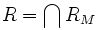$R = \bigcap R_M$
Let$a \in \bigcap R_M$. Let$I$ be the ideal comprising those$x \in R$ for which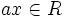$ax \in R$.$I$ is essentially the ideal of all possible denominators of fractions for$a$ in terms of elements of$R$.
The claim is that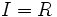$I = R$. Suppose not. Then$I$ is a proper ideal of$R$,and since every proper ideal is contained in a maximal ideal, we can find a maximal ideal$M$ containing$I$. But since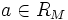$a \in R_M$,$a$ can be written as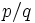$p/q$ where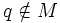$q \notin M$. Clearly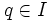$q \in I$, and this contradicts$I \le M$.